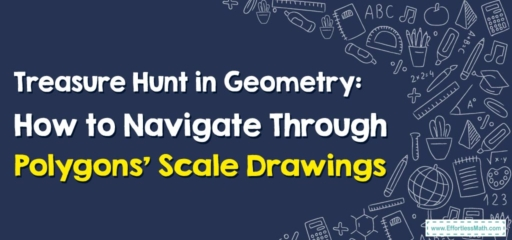# Treasure Hunt in Geometry: How to Navigate Through Polygons’ Scale Drawings

Today, our treasure map leads us through the labyrinth of geometry and scale drawings, specifically involving polygons. Get ready to decipher clues and use your math compass as we embark on this exciting journey!## 1. Unearthing the Clues: Understanding Polygons and Scale Drawings

Before we venture forth, let’s make sure we understand our tools and clues:

• Polygons: These are flat shapes with straight sides. Triangles, quadrilaterals, pentagons, and hexagons are all examples of polygons.
• Scale Drawings: These are representations of an object where the dimensions are proportional to the actual dimensions but at a reduced or increased size.

## 2. The Treasure Hunt: Polygons’ Scale Drawings

Now that we’re equipped, let’s embark on our treasure hunt!

### Adventure Guide: Navigating Through Polygons’ Scale Drawings

#### Step 1: Decipher the Scale

The first clue in our hunt is to understand the scale of the drawing. This scale tells us how the dimensions in the drawing compare to the actual dimensions of the polygon.

#### Step 2: Use the Scale

Once we have the scale, we use it to find missing dimensions or to create a scale drawing. Remember, every side length in the drawing is the actual side length times the scale factor.

#### Step 3: Apply to the Polygon

Finally, we apply our scale to the specific polygon in question. Whether it’s a triangle, a rectangle, or a complex dodecagon, our scale factor will help us navigate through.

For example, if we have a scale factor of $$2$$ and a drawing of a triangle with sides $$3\ cm, 4\ cm$$, and $$5\ cm$$, what are the lengths of the actual triangle’s sides?

1. Decipher the Scale: Our scale factor is $$2$$, meaning the actual object is twice as large as our drawing.
2. Use the Scale: Multiply each side by the scale factor. So, our actual triangle’s sides are $$3\ cm\times 2 = 6\ cm, 4cm\times 2 = 8\ cm$$, and $$5\ cm\times 2 = 10\ cm$$.

We’ve successfully navigated our way through polygons’ scale drawings! Remember, adventurers, geometry is a vast world filled with numerous treasures waiting to be discovered. Until our next math adventure, keep your compass handy and your curiosity alive!

### What people say about "Treasure Hunt in Geometry: How to Navigate Through Polygons’ Scale Drawings - Effortless Math: We Help Students Learn to LOVE Mathematics"?

No one replied yet.

X
30% OFF

Limited time only!

Save Over 30%

SAVE $5 It was$16.99 now it is \$11.99